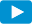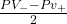Notes for Level I CFA® Program

R54 Understanding Fixed-Income Risk and Return

3.6. Money Duration of a Bond and the Price Value of a Basis PointWatch VideoThe money duration of a bond is a measure of the price change in units of the currency in which the bond is denominated, given a change in annual yield to maturity.

Money Duration = AnnModDur x PVFULL

ΔPVFULL≈ -MoneyDur x Δyield

Consider a bond with a par value of $100 million. The current yield to maturity (YTM) is 5% and the full price is$102 per $100 par value. The annual modified duration of this bond is 3. the money duration can be calculated as the annual modified duration (3) multiplied by the full price ($102 million): 3 x $102 million =$306 million. If the YTM rises by 1% (100 bps) from 5% to 6% the decrease in value will be approximately $306 million x 1% =$3.06 million. If the YTM rises by 0.1% (10 bps), the decrease in value will be $306 million x 0.1% =$0.306 million.

An important measure which is related to money duration is the price value of a basis point (PVBP). The PVBP is an estimate of the change in the full price given a 1 bp change in the yield-to-maturity. The formal equation is given below.

PVBP =where PV_ and PV+ are full prices calculated by decreasing and increasing the YTM by 1 basis point.

A quick way of calculating the price value of a basis point is to take the money duration and multiply by 0.0001. For example, if the money duration of a portfolio is $200,000 the price value of a basis point is$200,000 x 0.0001 = $20. (1 bp = 0.01% = 0.0001) Example 13: Calculating money duration of a bond A life insurance company holds a USD 1 million (par value) position in a bond that has a modified duration of 6.38. The full price of the bond is 102.32 per 100 of face value. 1. Calculate the money duration for the bond. 2. Using the money duration, estimate the loss for each 10 bps increase in the yield-to-maturity. Solution: 1. First calculate the full price of the bond:$1,000,000 x 102.32% = $1,023,200. The money duration for the bond is: 6.38 ×$1,023,200 = $6,528,000. 2. 10 bps corresponds to 0.10% = 0.0010. For each 10 bps increase in the yield-to-maturity, the loss is estimated to be:$6,528,000 × 0.0010 = $6,528.02. Example 14: Calculating PVBP for a bond Consider a$100, five-year bond that pays coupons at a rate of 10% semi-annually. The YTM is 10% and it is priced at par. The modified duration of the bond is 3.81. Calculate the PVBP for the bond.

Solution:

Money duration = $100 x 3.81 =$381.00

PVBP = $381 x 0.0001 =$0.0381